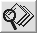poisplot Poissonness plot for discrete distributions poisplot

# Visualizing Categorical Data: poisplot

\$Version: 1.4 (31 Aug 2006)
Michael Friendly
York University

## The poisplot macro (get poisplot.sas)

### Poissonness plot for discrete distributions

The POISPLOT macro constructs a ``Poissonness plot'' for determining if discrete data follows the Poisson distribution. The plot has a linear relation between the count metameter `n(k)` and the basic count, k, when the distribution is Poisson. An influence plot displays the effect of each observed frequency on the choice of the Poisson parameter, lambda.

## Usage

The POISPLOT macro is called with keyword parameters. The COUNT= and FREQ= parameters are required. The arguments may be listed within parentheses in any order, separated by commas. For example:
```  %poisplot(count=Deaths,freq=corpsyrs, plot=dist);
```

### Parameters

Default values are shown after the name of each parameter.
DATA=
The name of the input data set [Default: `DATA=_LAST_`]
COUNT=
The name of the basic count variable
FREQ=
The name of the variable giving the number of occurrences of COUNT.
LABEL=
Label for the horizontal (COUNT=) variable. If not specified the variable label for the COUNT= variable in the input data set is used.
LAMBDA=
Trial value of the Poisson parameter lambda to level the plot. If `LAMBDA=0 `(the default) the plot is not levelled.
Z=
Multiplier for error bars [Default: `Z=1.96`]
PLOT=
What to plot: DIST and/or INFL [Default: `PLOT=DIST INFL`]
HTEXT=
Height of text labels [Default: `HTEXT=1.4`]
OUT=
The name of the output data set [Default: `OUT=POISPLOT`]
NAME=
Name of the graphics catalog entry [Default: `NAME=POISPLT`]

### Example

```%include vcd(poisplot);        *-- or include in an autocall library;
data horskick;
input deaths corpsyrs;
label deaths='Number of Deaths'
corpsyrs='Number of Corps-Years';
cards;
0    109
1     65
2     22
3      3
4      1
;
%poisplot(count=Deaths,freq=corpsyrs, plot=dist);
```